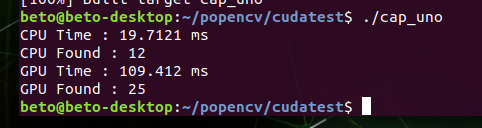# CUDA is so slow

Hi everybody, I’m newbie in Jetson nano & CUDA. I’m using Opencv 4.5 & Cuda 10.2, I’ve installed and compiled them succesufully in my nano board.
Just testing a code to learn about using CUDA in OpenCv, and speed up the task, but I found using cuda I get low fps than without using it. Any ideas what am I doing wrong ? This is my test code:

Hi,
There may be conversion between cv::Mat and cv::gpu::gpuMat. This is additional memory copy and impacts performance. It is better to have buffers in cv::gpu::gpuMat and avoid converting to cv::Mat.

Hi DaneLLL, thank you for your answer, but sorry, I can’t understand what you mean… :(
Testing the fps of that code, I found that without uploading & downloading before to apply cuda filters, does not impact enough in the final performance, and without using cuda standard opencv filters are fastest anyway.
I’m a begginer with alll of that, and I use similar code than in examples found by there. If I use only one filter in Preprocessing, seems that all works fast using cuda, but when I add all the tasks (convert to gray, blur, canny and dilate) the final performance is about four times slower in cuda. Excuse me if I’m not enough clear explaining my doubts… Please I’m just learning, and can’t found any answer google about this in web, so more help is wellcome. Thank you again. Alberto.

Hi, me again…Still I’m stuck with this… :(
Now I’ve tried and opencv example that is in my “/usr/share/opencv4/samples/gpu” folder
That code is below: (I’ve just only change the name of the file, it is for test houghlines using cpu and gpu)
When I run that code I recieve:As you can see that’s my issue, gpu time is greather than cpu time.
Please, has someone an idea what is happening with my Jetson nano board ?
Thank you. Alberto.

``````#include <cmath>
#include <iostream>
#include "opencv2/core.hpp"
#include <opencv2/core/utility.hpp>
#include "opencv2/highgui.hpp"
#include "opencv2/imgproc.hpp"
#include "opencv2/cudaimgproc.hpp"

using namespace std;
using namespace cv;
using namespace cv::cuda;

static void help()
{
cout << "This program demonstrates line finding with the Hough transform." << endl;
cout << "Usage:" << endl;
cout << "./cap_uno <image_name>, Default is /data/pic1.png\n" << endl;
}

int main(int argc, const char* argv[])
{
const string filename = argc >= 2 ? argv : "data/pic1.png";

if (src.empty())
{
help();
cout << "can not open " << filename << endl;
return -1;
}

Mat dst_cpu;
Mat dst_gpu = dst_cpu.clone();

vector<Vec4i> lines_cpu;
{
const int64 start = getTickCount();

cv::HoughLinesP(mask, lines_cpu, 1, CV_PI / 180, 50, 60, 5);

const double timeSec = (getTickCount() - start) / getTickFrequency();
cout << "CPU Time : " << timeSec * 1000 << " ms" << endl;
cout << "CPU Found : " << lines_cpu.size() << endl;
}

for (size_t i = 0; i < lines_cpu.size(); ++i)
{
Vec4i l = lines_cpu[i];
line(dst_cpu, Point(l, l), Point(l, l), Scalar(0, 0, 255), 3, LINE_AA);
}

GpuMat d_lines;
{
const int64 start = getTickCount();

Ptr<cuda::HoughSegmentDetector> hough = cuda::createHoughSegmentDetector(1.0f, (float) (CV_PI / 180.0f), 50, 5);

hough->detect(d_src, d_lines);

const double timeSec = (getTickCount() - start) / getTickFrequency();
cout << "GPU Time : " << timeSec * 1000 << " ms" << endl;
cout << "GPU Found : " << d_lines.cols << endl;
}
vector<Vec4i> lines_gpu;
if (!d_lines.empty())
{
lines_gpu.resize(d_lines.cols);
Mat h_lines(1, d_lines.cols, CV_32SC4, &lines_gpu);
}

for (size_t i = 0; i < lines_gpu.size(); ++i)
{
Vec4i l = lines_gpu[i];
line(dst_gpu, Point(l, l), Point(l, l), Scalar(0, 0, 255), 3, LINE_AA);
}

imshow("source", src);
imshow("detected lines [CPU]", dst_cpu);
imshow("detected lines [GPU]", dst_gpu);
waitKey();

return 0;
}type or paste code here
``````

Hi,

It looks like this is a duplicate of the topic 218047.
Let’s discuss the following on the above topic instead.

Thanks.

This topic was automatically closed 14 days after the last reply. New replies are no longer allowed.